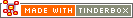sqrt(argument)Operator Type: Mathematical Operator Scope of Action: Item

sqrt(argument)

sqrt() computes the square root of its argument.

 Up: Mathematical operators Previous: sin(argument) Next: tan(argument)

[Last updated: 14 Dec 2009, using v5.0]